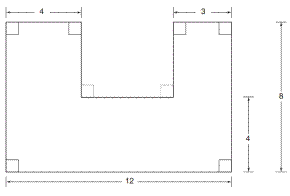Chapter 8.1, Problem 18E### Elementary Geometry for College St...

6th Edition
Daniel C. Alexander + 1 other
ISBN: 9781285195698

#### Solutions

Chapter
Section### Elementary Geometry for College St...

6th Edition
Daniel C. Alexander + 1 other
ISBN: 9781285195698
Textbook Problem
1 views

# In Exercises 9 to 18, find the areas of the figures shown or described.To determine

To find:

The area of the given figure.

Explanation

1) Approach:

Finding the area of the figure is followed by identifying the figure and find area with the suitable geometry formulae.

2) Calculation:

Given,

The given figure as rectangle in the form is given by

The area of the given figure A=(A1)area of a larger rectangle (A2)area of a smaller rectangle

Area of larger rectangle A1=l1 w1 unit2

Length of larger rectangle l1=12

Width of larger rectangle w1=8

A1=(12)(8)

A1=96 unit2

### Still sussing out bartleby?

Check out a sample textbook solution.

See a sample solution

#### The Solution to Your Study Problems

Bartleby provides explanations to thousands of textbook problems written by our experts, many with advanced degrees!

Get Started

#### Find the limit. limx/41tanxsinxcosx

Single Variable Calculus: Early Transcendentals, Volume I

#### 9. List four mortgage loan closing costs. (14-4)

Contemporary Mathematics for Business & Consumers

#### If x = ln( sec + tan ), show that sec = cosh x.

Single Variable Calculus: Early Transcendentals